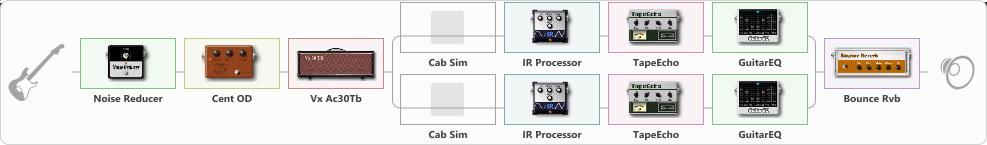# Rustam

Discussion in 'ToneLib-GFX presets' started by Assymmetry, Mar 6, 2023.

1. Rustam

Preset name: Driven Power Solo

Effects chain:Effect: "Noise Reducer" (Dynamics / Filter), active - "yes"
{
"Sens" = 100
"Mode" = Soft
}

Effect: "Cent OD" (Overdrive / Distortion), active - "yes"
{
"Drive" = 75
"Tone" = 64
"Level" = 100
}

Effect: "Vx Ac30Tb" (Amp simulators), active - "yes"
{
"Gain" = 70
"Bass" = 61
"Middle" = 36
"Treble" = 67
"Presence" = 66
"Master" = 100
"Level (dB)" = 0
}

Effect: "Splitter" (Dynamics / Filter)
{
"A-Bypass" = Off
"A-Pan" = -100
"A-Level" = 55
"B-Bypass" = Off
"B-Pan" = 100
"B-Level" = 55
"Width" = 36

'A' branch:
{

Effect: "Cab Sim" (Cabinets), active - "no"
{
"Model" = 2x12" Silver Bell
"Level (dB)" = 0
}

Effect: "IR Processor" (Cabinets), active - "yes"
{
"IR" = 7
"Low Cut (Hz)" = 0
"Hi Cut (kHz)" = 20.0
"Mix" = 100
"Level (dB)" = 0
}

Effect: "TapeEcho" (Delay), active - "yes"
{
"Time" = Note sync, 1/8 Dotted
"Feedback" = 34
"Tone" = 65
"LoDamp" = 19
"Mix" = 33
}

Effect: "GuitarEQ" (Dynamics / Filter), active - "yes"
{
"160 Hz" = 0
"400 Hz" = 0
"800 Hz" = 0
"1.6 kHz" = 0
"3.2 kHz" = 0
"6.4 kHz" = -3
"12 kHz" = 0
"Level (dB)" = 0
}
}
'B' branch:
{

Effect: "Cab Sim" (Cabinets), active - "no"
{
"Model" = 1x12" Blue Bell
"Level (dB)" = 0
}

Effect: "IR Processor" (Cabinets), active - "yes"
{
"IR" = 15
"Low Cut (Hz)" = 0
"Hi Cut (kHz)" = 19.5
"Mix" = 100
"Level (dB)" = 0
}

Effect: "TapeEcho" (Delay), active - "yes"
{
"Time" = Note sync, 1/16 Dotted
"Feedback" = 70
"Tone" = 65
"LoDamp" = 19
"Mix" = 37
}

Effect: "GuitarEQ" (Dynamics / Filter), active - "yes"
{
"160 Hz" = 0
"400 Hz" = 0
"800 Hz" = 0
"1.6 kHz" = 0
"3.2 kHz" = 0
"6.4 kHz" = -3
"12 kHz" = 0
"Level (dB)" = 0
}
}
}

Effect: "Bounce Rvb" (Reverberation), active - "yes"
{
"Time" = 2.7
"PreDelay" = 32
"LoDamp" = 16
"HiDamp" = 26
"Mix" = 30
}

Note: You will need to download and install the ToneLib-GFX software to use the preset.

#### Attached Files:

• ###### Rustam.tlgfx
File size:
3.9 KB
Views:
3,164
YurijPa, _Yorick420 and The MASK like this.